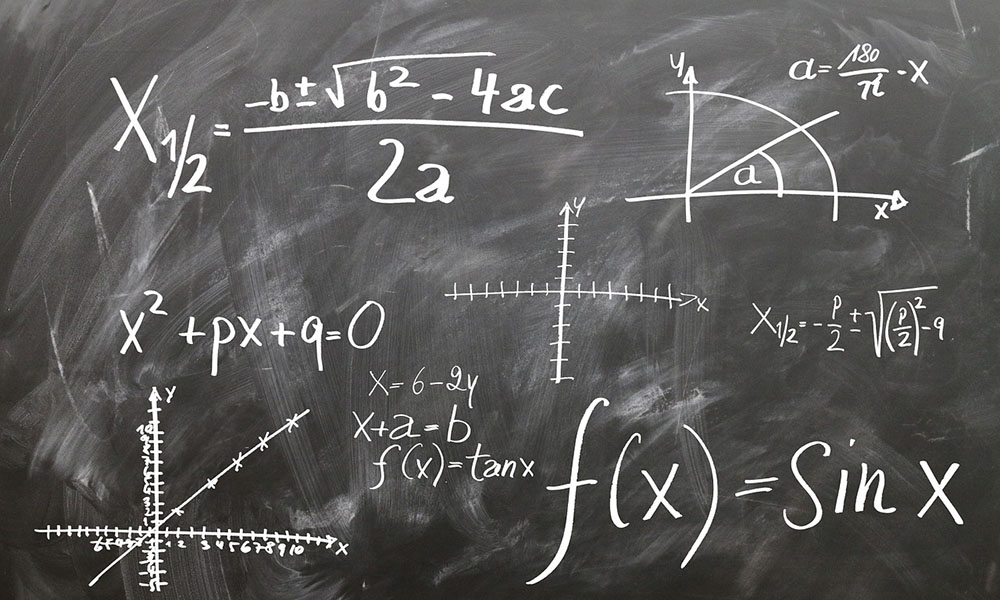# Planck Units

Having discovered the fundamental constant h, which relates energy to frequency, Max Planck realized that he had all the elements required to calculate fundamental units of length, mass, time, charge and temperature.

The calculations were simple to the point of being trivial. All Planck needed to do was to play around with the fundamental constants until they combined in such a way that the calculated values come out with a single dimension. The combination that yielded a number denoted in meters became the Planck length. The combination that resulted in a number denoted in seconds became the Planck time. Planck mass is similarly denoted in gram, charge in Coulomb and temperature in Kelvin. Together, they became known as Planck units.

The thinking was clear and simple. If fundamental constants are truly fundamental, then any unit derived from these constants must also be fundamental. These units must tell us something fundamental about the universe and its components. For example, there should be something in the universe that has the radius, diameter or circumference of a Planck length. Similarly, there should be things in the universe that correspond to Planck’s calculated units of mass, time, charge and temperature.

In this respect, it’s interesting to note how the Planck length and time fit with the ideas of distance and time laid out in my proposed physics. Planck length is calculated from Planck’s constant h, the light speed c and the gravitational constant G. This yields a very small number. In my proposed physics, the smallest possible ruler we can use to measure distance is the electron, a very small particle.

Planck time is in turn the Planck length divided by the speed of light. This is identical in form to my proposed definition of time, where the smallest possible time unit is the time that it takes a photon to cross an electron.

The strict particle model proposed in my book suggests that there is something special about the electron in that it represents the smallest ruler possible as well as the smallest clock. It seems then that the Planck length is in fact the radius, diameter or circumference of an electron.

This being said, I’m skeptical to the accuracy of the calculated Planck length. I’m not convinced that the gravitational constant G is in fact a constant. Gravity is in my book an imbalance in the electric force, and any constant related to gravity should therefore be a simple proportion of the electric constant. Planck units that involve the constant G in their calculation are in my view suspect.

However, this does not take away the genius of Planck’s units. They point to something fundamental, and can therefore help us in our understanding of how everything hangs together. Suspect units with very strange values can be identified, giving us a clue to where our thinking may have gone wrong.

#### This Post Has 0 Comments

This site uses Akismet to reduce spam. Learn how your comment data is processed.